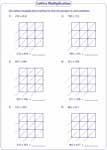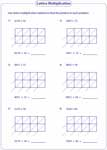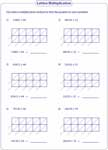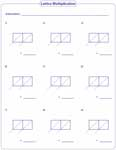# Lattice Multiplication: Worksheets and Grids

Lattice multiplication is a simple technique that breaks long multiplication process into smaller steps. This page includes printable worksheets for 3rd grade, 4th grade, and 5th grade children on multiplying numbers from single digit to four digit in different combinations. Lattice multiplication grids (templates) are also included for teachers and homeschool moms. Delve into some of these worksheets for free!

Lattice Multiplication: 2-digit and single-digit

Grade 3 worksheets tasks kids to multiply 2-digit numbers by single-digit numbers using lattice multiplication.Lattice Multiplication: 2-digit and 2-digit

Use lattice multiplication to multiply 2-digit multiplicands.Lattice Multiplication: 3-digit with 2-digit

Apply lattice method to multiply 3-digit and 2-digit using 2 x 3 grids.Lattice Multiplication: 3-digit and 3-digit

Use lattice method to multiply 3-digit multiplicands in these pdf worksheets.Multiplying 4-digit Numbers

The printable worksheets for grade 4 and grade 5 kids consists of lattice multiplication on 4-digit by 2-digit, 4-digit by 3-digit and 4-digit by 4-digit.4-digit and 2-digit:

4-digit and 3-digit:

4-digit and 4-digit:

Multiplying 5-digit Numbers

Exercises in these pdf worksheets have multiplication problems on 5-digit with 2 or 3 digit multiplicands.5-digit and 2-digit:

5-digit and 3-digit:

Lattice Multiplication Grids: Teacher Template

Lattice multiplication templates help teachers, homeschooling moms and tutors to create their own worksheets. The lattice grids are available in different sizes. Choose, download, set your own questions and assign them to the kids.Lattice Multiplication Grid: 2 x 2

Lattice Multiplication Grid: 2 x 3

Lattice Multiplication Grid: 3 x 3

Lattice Multiplication Grid: 2 x 4

Lattice Multiplication Grid: 3 x 4

Lattice Multiplication Grid: 4 x 4

Lattice Multiplication Grid: 2 x 5

Lattice Multiplication Grid: 3 x 5

Related Worksheets